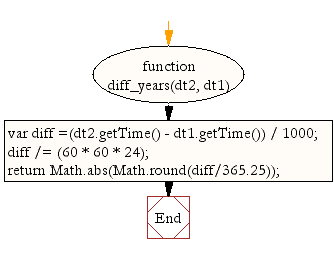# JavaScript: Get time differences in years between two dates

## JavaScript Datetime: Exercise-49 with Solution

Write a JavaScript function to get time differences in years between two dates.

Test Data :
dt1 = new Date("June 13, 2014 08:11:00");
dt2 = new Date("October 19, 2017 11:13:00");
console.log(diff_years(dt1, dt2));
3

Sample Solution:-

HTML Code:

``````<!DOCTYPE html>
<html>
<head>
<meta charset="utf-8">
<title>JavaScript function to get time differences in years between two dates</title>
</head>
<body>

</body>
</html>
```
```

JavaScript Code:

``````function diff_years(dt2, dt1)
{

var diff =(dt2.getTime() - dt1.getTime()) / 1000;
diff /= (60 * 60 * 24);
return Math.abs(Math.round(diff/365.25));

}

dt1 = new Date(2014,10,2);
dt2 = new Date(2014,10,11);
console.log(diff_years(dt1, dt2));

dt1 = new Date("June 13, 2014 08:11:00");
dt2 = new Date("October 19, 2017 11:13:00");
console.log(diff_years(dt1, dt2));
```
```

Sample Output:

```0
3
```

Flowchart:Live Demo:

See the Pen JavaScript - Get time differences in years between two dates-date-ex-49 by w3resource (@w3resource) on CodePen.

Improve this sample solution and post your code through Disqus

What is the difficulty level of this exercise?

﻿

## JavaScript: Tips of the Day

Converts the given array elements into <li> tags and appends them to the list of the given id.

Example:

```const tips_arrayToHtmlList = (arr, listID) =>(el => ((el = document.querySelector('#' + listID)),(el += arr.map(item => '<li>\${item}</li>').join(''))))();
console.log(tips_arrayToHtmlList(['item 1', 'item 2'], 'myListID'));
```

Output:

```"null\${item}\${item}"
```